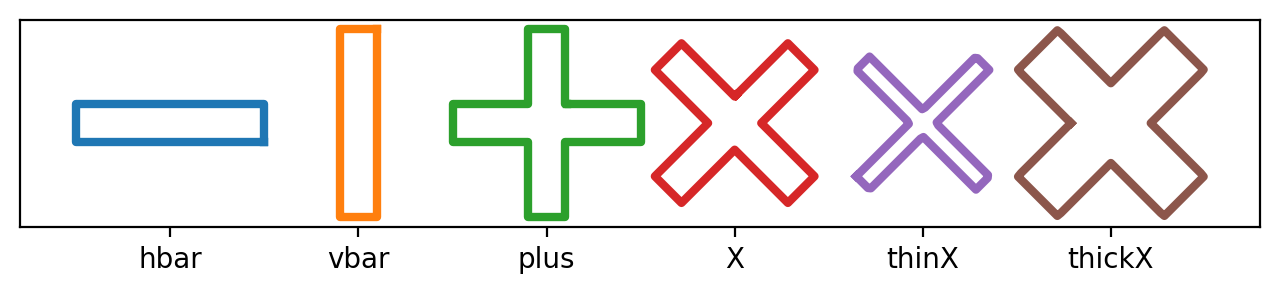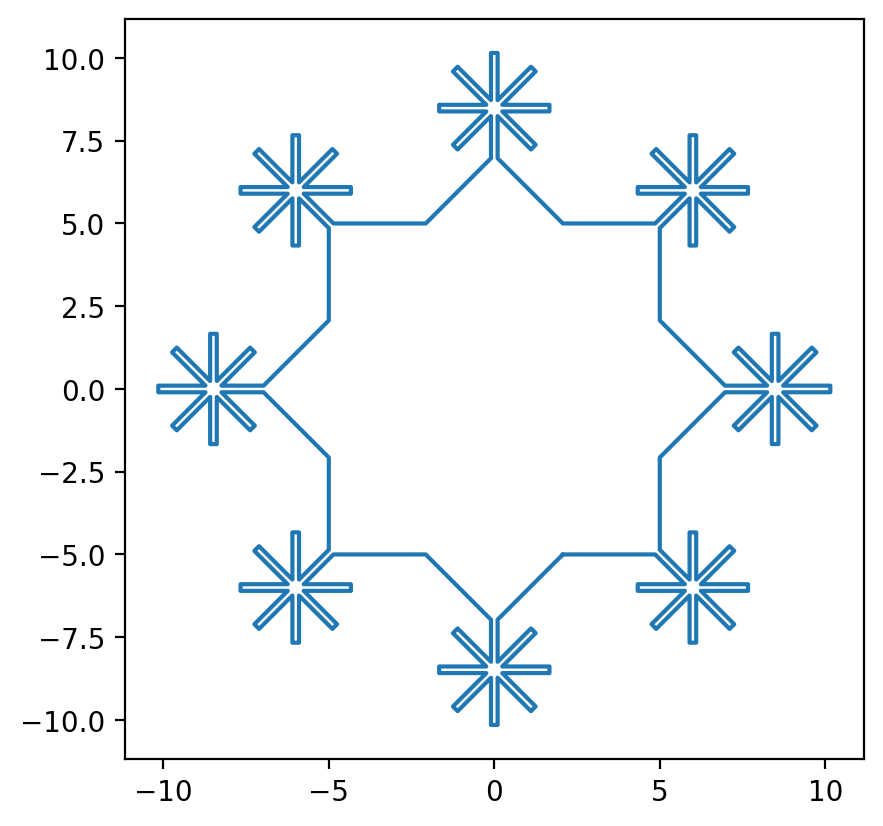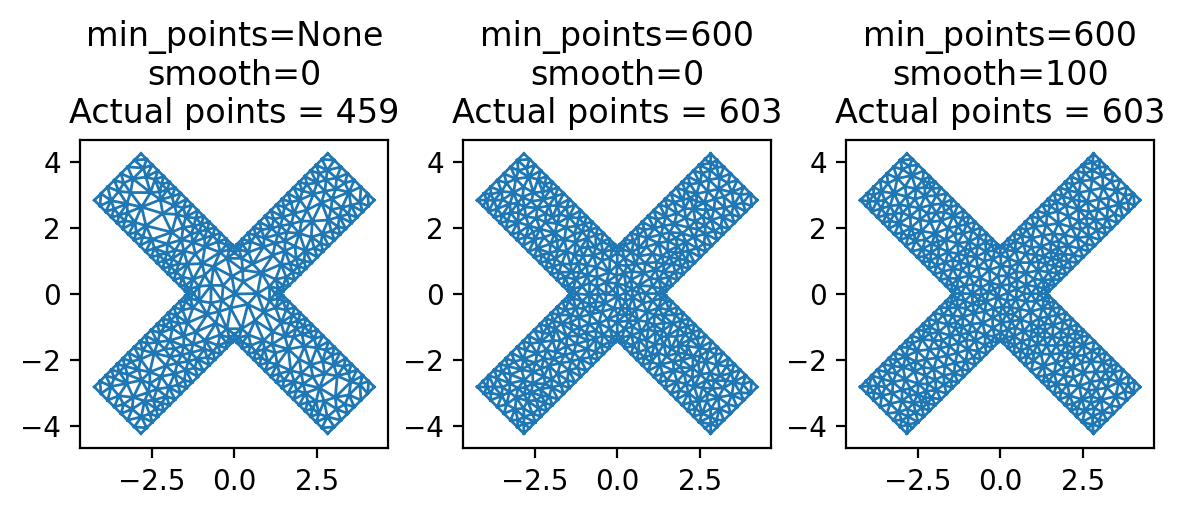# Working with polygons

At the core of each pyTDGL simulation is an instance of the tdgl.Device class, which represents the superconducting structure to be modeled. A Device is composed a Layer that lies in a plane parallel to the $$x-y$$ plane (at position layer.z0) and has a specified thickness $$d$$, coherence length $$\xi$$ and London penetration depth $$\lambda$$. The layer contains superconducting film, which can contain zero or more holes. Films and holes are represented by instances of the tdgl.Polygon class, which defines a 2D polygonal region.

:

%config InlineBackend.figure_formats = {"retina", "png"}
%matplotlib inline

import numpy as np
import matplotlib.pyplot as plt

import tdgl


A Polygon is defined by a collection of (x, y) coordinates specifying its vertices; the vertices are stored as an n x 2 numpy.ndarray called polygon.points.

:

# Define the initial geometry: a rectangular box specified as an np.ndarray
width, height = 10, 2
points = tdgl.geometry.box(width, height)
print(f"type(points) = {type(points)}, points.shape = {points.shape}")

type(points) = <class 'numpy.ndarray'>, points.shape = (100, 2)

:

# Create a Polygon representing a "horizontal bar", hbar
hbar = tdgl.Polygon(points=points)
hbar.polygon

:The object passed to tdgl.Polygon(points=...) can be of any of the following:

• An n x 2 array-like object, for example an np.ndarray or a list of (x, y) coordinates

• An existing tdgl.Polygon instance (in which case, the new object will be a copy of the existing one)

• An instance of LineString, LinearRing, or Polygon from the shapely package

:

(
tdgl.Polygon(points=hbar.points)
== tdgl.Polygon(points=hbar.polygon)
== tdgl.Polygon(points=hbar)
== hbar.copy()
== hbar
)

:

True


Every instance of tdgl.Polygon has a property, instance.polygon, which returns a corresponding shapely Polygon object. Among other things, this is usefuly for quickly visualizing polygons.

There are several methods for transforming the geometry of a single Polygon:

• polygon.translate(dx=0, dy=0)

• polygon.rotate(degrees, origin=(0, 0))

• polygon.scale(xfact=1, yfact=1, origin=(0, 0))

• polygon.buffer(distance, ...)

There are also three methods for combining multiple Polygon-like objects:

• polygon.union(*others): logical union of polygon with each object in the iterable others

• See also: tdgl.Polygon.from_union([...])

• polygon.intersection(*others): logical intersection of polygon with each object in the iterable others

• See also: tdgl.Polygon.from_intersection([...])

• polygon.difference(*others): logical difference of polygon with each object in the iterable others

• See also: tdgl.Polygon.from_difference([...])

Note that the elements of the iterable others can be of any type that can be passed in to tdgl.Polygon(points=...) (see above).

:

# Copy hbar and rotate the copy 90 degrees counterclockwise
vbar = hbar.rotate(90)
vbar.polygon

::

# Create a new Polygon that is the union of hbar and vbar: "+"
plus = hbar.union(vbar)
# # The above is equivalent to either of the following:
# plus = vbar.union(hbar)
# plus = tdgl.Polygon.from_union([hbar, vbar])
plus.polygon

::

# Rotate the "+" by 45 degrees to make an "X"
X = plus.rotate(45)
X.polygon

::

# Create a new polygon with all edges offset (eroded) by a distance of -0.5
thinX = X.buffer(-0.5)
thinX.polygon

::

# Create a new polygon with all edges offset (expanded) by a distance of 0.5
thickX = X.buffer(0.5)
thickX.polygon

::

polygons = [hbar, vbar, plus, X, thinX, thickX]
labels = ["hbar", "vbar", "plus", "X", "thinX", "thickX"]

fig, ax = plt.subplots(figsize=(8, 1.5))

for i, polygon in enumerate(polygons):
polygon.translate(dx=width * i).plot(ax=ax, linewidth=3)

ax.set_xticks([width * i for i, _ in enumerate(labels)])
ax.set_xticklabels(labels)
_ = ax.set_yticks([]):

X.union(plus).polygon

::

X.intersection(plus).polygon

:Using the methods demonstrated above, intricate geometries can be constructed from simple building blocks in just a few lines of code.

:

size = 10

hbar = tdgl.Polygon(points=tdgl.geometry.box(size / 3, size / 50))
plus = hbar.union(hbar.rotate(90))
star = plus.union(plus.rotate(45))

star_dx = 1.2 * size * np.sqrt(2) / 2

snowflake = (
tdgl.Polygon(points=tdgl.geometry.box(size, size))
.rotate(45)
.union(
*(star.translate(dx=star_dx).rotate(degrees) for degrees in [0, 90, 180, 270])
)
)
snowflake = snowflake.union(snowflake.rotate(45))

:

ax = snowflake.plot():

print(f"Polygon area: snowflake.area = {snowflake.area:.3f}")
print(f"Polygon width and height: snowflake.extents = {snowflake.extents}")

Polygon area: snowflake.area = 136.622
Polygon width and height: snowflake.extents = (20.303896081810475, 20.303896081810475)


## Meshing Polygons

Individual polygons can be meshed using the Polygon.make_mesh() method.

:

setups = [
dict(min_points=None, smooth=0),
dict(min_points=350, smooth=0),
dict(min_points=350, smooth=100),
]

fig, axes = plt.subplots(1, len(setups), figsize=(2 * (len(setups) + 0.5), 2))

for ax, options in zip(axes, setups):
# Generate a mesh with the specified options
mesh = X.make_mesh(**options)

# Plot the mesh
ax.set_aspect("equal")
title = [f"{key}={value!r}" for key, value in options.items()]
title.append(f"Actual points = {mesh.x.shape}")
ax.triplot(mesh.x, mesh.y, mesh.elements, lw=1)
ax.set_title("\n".join(title))

Constructing Voronoi polygons: 100%|██████████| 459/459 [00:00<00:00, 2767.94it/s]
Constructing Voronoi polygons: 100%|██████████| 459/459 [00:00<00:00, 2783.49it/s]
Constructing Voronoi polygons: 100%|██████████| 459/459 [00:00<00:00, 2689.26it/s]:

tdgl.version_table()

:

SoftwareVersion
tdgl0.5.1
Numpy1.25.2
SciPy1.10.1
matplotlib3.8.0
jaxNone
numba0.58.0
IPython8.15.0
Python3.10.12 (main, Jul 27 2023, 08:44:48) [GCC 11.3.0]
OSposix [linux]
Number of CPUsPhysical: 1, Logical: 2
BLAS InfoOPENBLAS
Fri Sep 22 01:27:52 2023 UTC
[ ]: